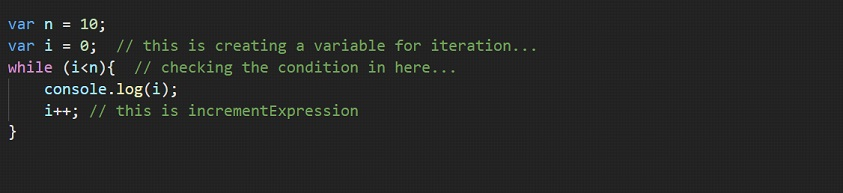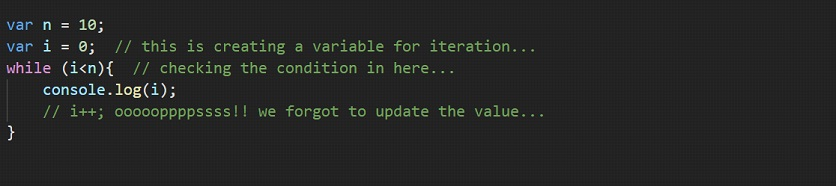# While Loop Javascript

Well, the while loop Javascript is another type of loop Javascript. Since this is a loop, we are doing the same thing here as well. Remember from the for loop in javascript, that we had created some variable for iteration, then there was some condition check, and then there comes some change in the value of the iteration variable.

## While Loop Javascript

In the for loop, we were doing all three things together in a bracket (after the for a keyword). Here as well, we are going to have these three things, but the syntax is going to be a little bit different here.

Have a look at the syntax below –As you can see, we have written the while keyword, and then inside the brackets after the while keyword, we are writing some conditions. This condition is going to be checked every time before entering the loop.

So, if the condition is true, then only the loop is going to execute. We are going to create a variable outside the while loop, using which, we are going to iterate through the loop.

We are going to update the values of the iteration variable inside the while loop.

If we want to translate what the while loop does, into a very understandable language, we can say that – while this(some condition) is true, do that(run some code).

Have a look at the below example, which is similar to the previous one(from the for loop), but this time, we are using the while loop to do the same thing –If we try to run the above program, we are going to get an output from 0 to 9. Once the value of i becomes 10, we run out of the loop, since the condition becomes false. Also, just to mention, you can also write i<10 directly here.

So, here you can see that we have some conditions. It can be any relevant condition. The while loop will execute till the condition is found to be true, and once the condition becomes false, we come out of the loop.

There is something called an infinite loop, which means that the loop will run forever. The loop will run forever only if the condition to run the loop never becomes false. So, let’s say that we initialized the variable for iteration, to a certain value, let’s say 0.

Now, we are also checking the condition, that the variable value should be less than 10. if this condition is true, then only we are going to log the value of the variable on the console. But, inside the while loop, we forgot to increment the variable(which takes us to the termination of the loop).

So, in the first iteration, the value the variable contains is 0, and the condition is true, so we are running the loop. Since we have not done an increment operation, the value from the variable is going to be 0 only. So, in the next iteration as well, the value is 0, and again, the condition is true, and again the loop will execute. Now, this goes on and on and on… This is the infinite loop. Have a look at the below program, where we have intentionally forgotten to increment the variable.If you try to run the program, you will get continuously 0 as an output. Alternatively, you can directly write true, where we write our condition. So, while (true), and then the code. If we do this, then also, our loop is going to run for infinity. You can try this as well…